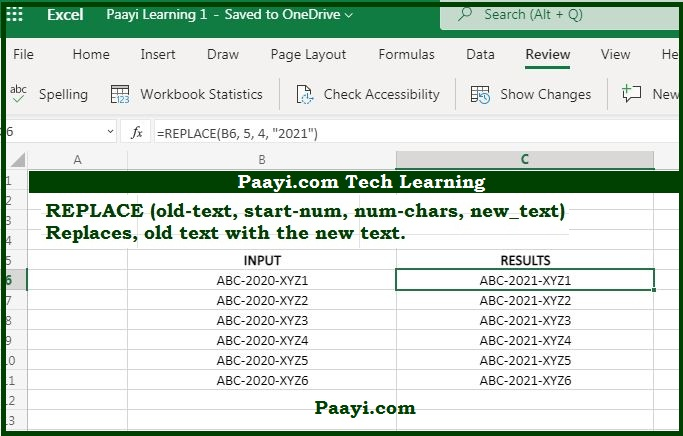# Learn How to use Microsoft Excel REPLACE Function

Written by | 0 Comments | 641 Views

In this article, you will learn how to use the MS Excel REPLACE function and its prime function in Microsoft Excel. You will also get to know the Microsoft Excel REPLACE function return value and syntax with the help of some examples.

## Microsoft Excel REPLACE Function

The primary purpose of the Microsoft Excel REPLACE function is to change the text based on location. That implies the REPLACE function replaces characters specified by the location of the text string provided with some other text string. You can understand this by the example given below, here:

The formula: = REPLACE("ABC123" 4,3, "678") will return the value - "ABC678".

### REPLACE Function Return Value

It will return the replaced text string.

### REPLACE Function Syntax

= REPLACE(old-text, start-num, num-chars, new-text)

Where the arguments:

• old-text: This is the text you want to replace.
• start-num: The starting location in the text to search.
• num-chars: The number of characters you want to replace.

new-text: The new text you want to replace with the old text.

## How to Use Microsoft Excel REPLACE Function?As we know, the REPLACE function is used to replace the old text string with the new text. You can understand this by the examples given below:

Example1:

= REPLACE("ABC123" 4, 3, "678") - This will return the value "ABC678".

Example 2: You can also remove the text, using the REPLACE function is given below, by specifying the empty string (" ") as new text:

= REPLACE("ABC", 1, 1, " ") - The return value will be "BC."